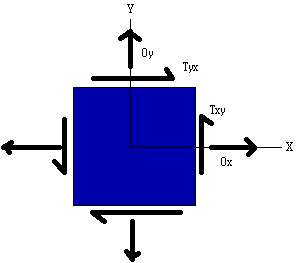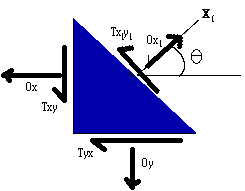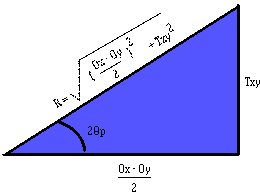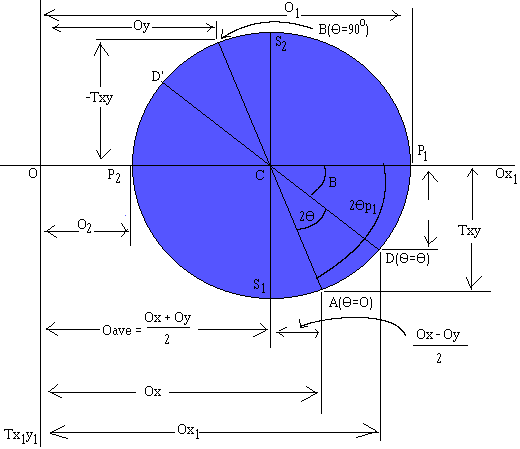UNDERSTANDING MOHR’S CIRCLE AND ITS APPLICATIONS IN ANALYZING PLANE STRESS

In an effort to gain the insight necessary to solve the problems myself, and to show those damned civil engineers that I can indeed grasp their useless abstractions (useless because there are a great number of higher-level mathematical techniques which could be used to solve these problems in a much more straight forward manner) I will now derive and explain Mohr's circle in relation to analyzing plane stresses.Examination of the normal (O) and the shear stresses (T) acting upon a cross-section of a larger body (figure to the left) allows us to analyze the stresses and strains on sections inclined with respect to this one (figure to the right.) By equilibrium we find that, via sign conventions,Txy = Tyx

which will simplify things as the derivation progresses. The stresses acting on the inclined X1, Y1 element (right) can be expressed in terms of the stresses on the X, Y element (left) by using Newtonian equations of equilibrium. Given that the force acting on an area is equal to the stress multiplied by the area, or

F = O A

we can resolve the forces acting on the left-hand and bottom faces of the section into orthogonal components acting in the X1 and Y1 directions. Let us denote the area of the left-hand side face (that is, the negative x face) as Ao. Then the normal and shear forces acting on that face are Ox Ao and Txy Ao. By trigonometric rules, the area of the bottom face of the inclined element (or negative y face) is Ao tan (theta), and the area of the inclined face (or positive X1 face) is Ao sec (theta). Now we can obtain two equations of equilibrium by summing the forces in the X1 and Y1 directions.

SUM(F[X1]) = 0 = Ox1Ao sec(theta) - OxAo cos(theta) - TxyAo sin(theta) - OyAo tan(theta)sin(theta) - TyxAo tan(theta)cos(theta)
SUM(F[Y1]) = 0 = Tx1y1Ao sec(theta) + OxAo sin(theta) - TxyAo cos(theta) - OyAo tan(theta)cos(theta) + TyxAo tan(theta)sin(theta)

Using the relationship Txy = Tyx and also simplifying and rearranging, we arrive at the following two equations:

Ox1 = Ox cos2(theta) + Oy sin2(theta) + 2Txy sin(theta)cos(theta)                                    (1)
Tx1y1 = - (Ox - Oy)sin(theta)cos(theta) + Txy[cos2(theta) - sin2(theta)]                              (2)

Equations (1) and (2) give the normal and shear stresses acting on the inclined plane in terms of the angle, theta, and the original stresses acting on the segment. For special cases when the angle is zero, we note that Ox1 = Ox and Tx1y1 = Txy, as we would expect.

Civil engineers claim that this can be "expressed in a more convenient form" by using certain trigonometric identities, but, honestly, convenience is a matter of opinion. Now, this is where I would have simply stopped. Right here is plenty of information I need to solve just about any plane stress problem thrown my way. It may be ugly, and it certainly could be simplified using some sort of vector or matrix notation, however, provided you can handle dealing with squared sines and cosines, and don't mind the derivation of and/or memorizing these equation, it's not too bad. BUT NOOOOOO! Some dumb-ass civil engineer was afraid of manipulating squared sines and cosines, and used the following identities:

cos2(theta) = (1/2)(1 + cos (2theta))            sin2(theta) = (1/2)(1 - cos(2theta))
sin(theta)cos(theta) = (1/2)sin(2theta)
to get the following results:
Ox1 = (Ox + Oy)/2 + {(Ox - Oy)/2}cos(2theta) + Txy sin(2theta)                        (3)
Tx1y1 = - {(Ox - Oy)/2}sin(2theta) + Txy cos(2theta)                                        (4)

Now, again, here is where I would have stopped. I would have a set of equations (slightly more complicated than (1) & (2) in my opinion) that is still within reason that I could derive on the fly and/or memorize. And some civil engineers were obviously fine with stopping here because they went ahead and named these equations the transformation equations for plane stress, because they transform the stress components from one set of axis to another.

Also, some slightly interesting things pop out of these equations. For one, when the original stresses are zero, except for Ox, then the element is said to be in uniaxial stress, meaning that it only is experiencing stress along one axis. In the uniaxial stress state, equations (3) and (4) reduce to

Ox1 = (Ox/2)[1 + cos(2theta)]                    Tx1y1 = (- Ox/2)[sin(2theta)]

Another special case is when both of the normal stresses are zero, and Txy is the only non-zero element. This case is called pure shear (not to be confused with pure evil, which is what most civil engineering teachers are) because it (drum roll, please) only involves shear. With Ox = 0 and Oy = 0, equations (3) and (4) reduce to

Ox1 = Txy sin(2theta)                Tx1y1 = Txy cos(2theta)

Lastly, there is the special case when the normal stresses are non-zero and the shear stresses are zero. This is termed biaxial stress. With Txy = 0, equations (3) and (4) become

Ox1 = (Ox + Oy)/2 + {(Ox - Oy)/2}cos(2theta)        Tx1y1 = -{(Ox - Oy)/2}sin(2theta)

PRINCIPAL STRESSES & MOHR'S CIRCLE

To find the maximum and minimum normal stresses throughout the entire range of angles, one can easily take the first derivative of (3) with respect to theta, set it to zero, and solve for the angle. This will give what is called the principal plane on which the principal stresses act. If this all sounds overly complicated... you're right! Why not just use the tried and true terminology "maximizing and minimizing the function" instead of inventing these two new terms with unrelated and unclear meaning? Well.... that's civil engineers for you.

dOx1/d(theta) = -(Ox - Oy)sin(2theta) + 2Txy cos(2theta) = 0
tan 2thetap = (2txy)/(Ox - Oy)                                    (5)

Where thetap defines the orientation of the principal planes, and its two values, differing by 180o, are called the principal angles.

Now is where we begin to get into the unnecessary jargon. All the excess baggage some engineer created to make it so that utilizing these relationships would not require higher math. This (and many other examples of engineer idioticy) most likely stems from the fact that most engineers slept through their higher level math classes, and suffer from acute mathematical insecurities (and probably rightly so.) It's these abstract constructions which attempt to simplify the work, yet ultimately make it more difficult for those of us more mathematically inclined, that really piss me off.If you represent equation (5) geometrically with a 90o triangle, (left), we can obtain general formulas for the principal stresses. First, we note that the hypotenuse of the triangle is,

R = SQR{ [(Ox - Oy)/2]2 + Txy2}                                (6)

The quantity R is defined as a positive number, and, like the other two sides of the triangle, has the completely meaningless units of "stress". From the triangle we obtain two additional relations:

cos(2thetap) = (Ox - Oy)/(2R)            sin(2thetap) = Txy/R                (7, 8)

Which is all very well and good, because it actually leads to the USEFUL equation for the general formula for the principal stresses:

O1,2 = (Ox + Oy)/2  +/- R                                        (9)

But such usefulness is short lived as we approach MOHR'S CIRCLE..... Actually, Mohr's circle isn't all that bad in many cases. It supplies its practitioners a clever and easy way to compute otherwise hairy moments of inertia, allows strain analyses to be handled quickly. However, in this case, its application seems to me a bit of a stretch, and what you wind up with is this hopelessly complicated graphical representation that seems so much more difficult than the original equations (3) and (4) that it's hardly worth the effort to learn at all. HOWEVER.... because certain bastich elements in the civil engineering department here at the U of A are requiring their students (many of whom, myself included, will NEVER use these relationships again after the class has ended) to use this technique in spite of the fact that we know of a perfectly valid and correct alternative.

The equations of Mohr's circle can be derived from the transformation equations (3) and (4). By simply rearranging the first equation, we find that the two expressions comprise the equation of a circle in parametric form.

Ox1 - (Ox + Oy)/2 = [(Ox - Oy)/2]cos(2theta) + Txy sin(2theta)                  (10)
Tx1y1 = - {(Ox - Oy)/2}sin(2theta) + Txy cos(2theta)                              (11)

To eliminate the 2theta parameter, we square each relationship and add the two equations together. This ultimately leads to (after simplification),

(Ox1 - {Ox + Oy}/2)2 + Tx1y12 = {(Ox - Oy)/2}2 + Txy2            (12)

However, by resubstitution of equation (6) and by recognizing that the average stress value between the X and Y axis, Oave, is,

Oave = (Ox + Oy)/2                                                    (12.a)
equation (12) can be simplified into the semi friendly equation of a circle in standard algebraic form,

(Ox1 - Oave)2 + Tx1y12 = R2            (13)

However, don't let  this nice looking equation for a circle fool you. Hidden in this simple equation are some of the most hairy, complicated, and down-right nasty relationships I think I have ever encountered. This makes my studies in the Frobenious theorem for solving differential equations with non-constant singular coefficients seem tame.With Ox, Oy, and Txy known, the procedure for constructing Mohr's circle is as follows:

1. Draw a set of coordinate axis with Ox1 and Tx1y1 (with T positive downwards. From now on, for simplicity, O and T will represent their respective axis.)
2. The center of the circle, by equation (13) is located at T=0 and O=Oave. Oave is nothing more than (12.a), so the center of the circle is located at:
C = (Ox + Oy)/2
3. Locate point A, representing the stress conditions on the X face of the normal oriented element (Figure 1, extreme top left, non-rotated section). Plot coordinates O = Ox, T = Txy. Here, it is important to note that at point A, the inclination angle, theta, is zero.
4. Locate point B, representing the stress conditions on the Y face of the normal oriented element (Figure 1, again, extreme top left, non-rotated section). Again, plot coordinates O = Oy, T = Txy. Note that this point, B, will be diametrically opposite from point A. Also note, that the angle of inclination at B, theta, will be 90o, as it could also be achieved on the X face by rotating it by 90o.
5. Draw a line from point A to point B through the center C. This line is a diameter of the circle.
6. Using point C as the center, draw Mohr's circle through points A and B. The circle will have a radius of R, which is the same R as in equation (6).
Now that you have Mohr's circle drawn, you can use it to analyze the problem. (Remember, that this method is every bit as valid as simply using equations (3) and (4) above, except it requires less mathematical skill, and many more memorized relationships.)

O1,2, representing the maximum and minimum normal stresses and their respective angles away from point A (where theta = 0o) can be found by simply looking at the O values when T = 0. In the drawing above, O1 represents the maximum, and O2 the minimum. Furthermore, Tmax/min, representing the maximum and minimum shear stresses and their respected angles can be found by locating the T values  when O = Oave. At this point, T is simply equal to the radius, R, or equation (6).

In addition to these helpful points, all other possible points for the shear and normal stresses can be found on this circle. In order to find another value of Ox, Oy for a given rotation, one must simply start at the A and B points (A representing the Ox value and B, the Oy value), and rotate in a positive theta direction (by the orientation shown above, this is in a counterclockwise direction, in keeping with  the right hand rule) for 2theta (from equations (3) and (4) above). The resulting points, D and D', will yield the Ox, Txy, and Oy, Txy (respectively) for that rotation.

As I have likely mentioned before (likely because, I can't really recall) to me this seems all very abstract and difficult to use. However, the aforementioned bastiches will be requiring this on my upcoming test, so I felt a need to more fully understand it. Granted, I still don't understand it as fully as I would hope, but it ought to be enough to get me through this one, insignificant little test.

P.S.: I apologize for my editorializing and opinionated presentation of this topic. I rarely do this when I analyze problems I don't understand (even when I do not like the method, such as the Lewis Dot structure). This time, however, I have some very strong feelings about my predicament. Also, in all fairness, if you were given the problem where O1 = O2 and Tmax = 0, i.e. the Mohr's circle was simply a little dot with R = 0, using the Mohr's circle method would arrive you at any and all answers much quicker than using equations (3) and (4). However, I don't think this extreme simplification of one special case warrants the abstraction being a required bit of knowledge for civil engineers. -->